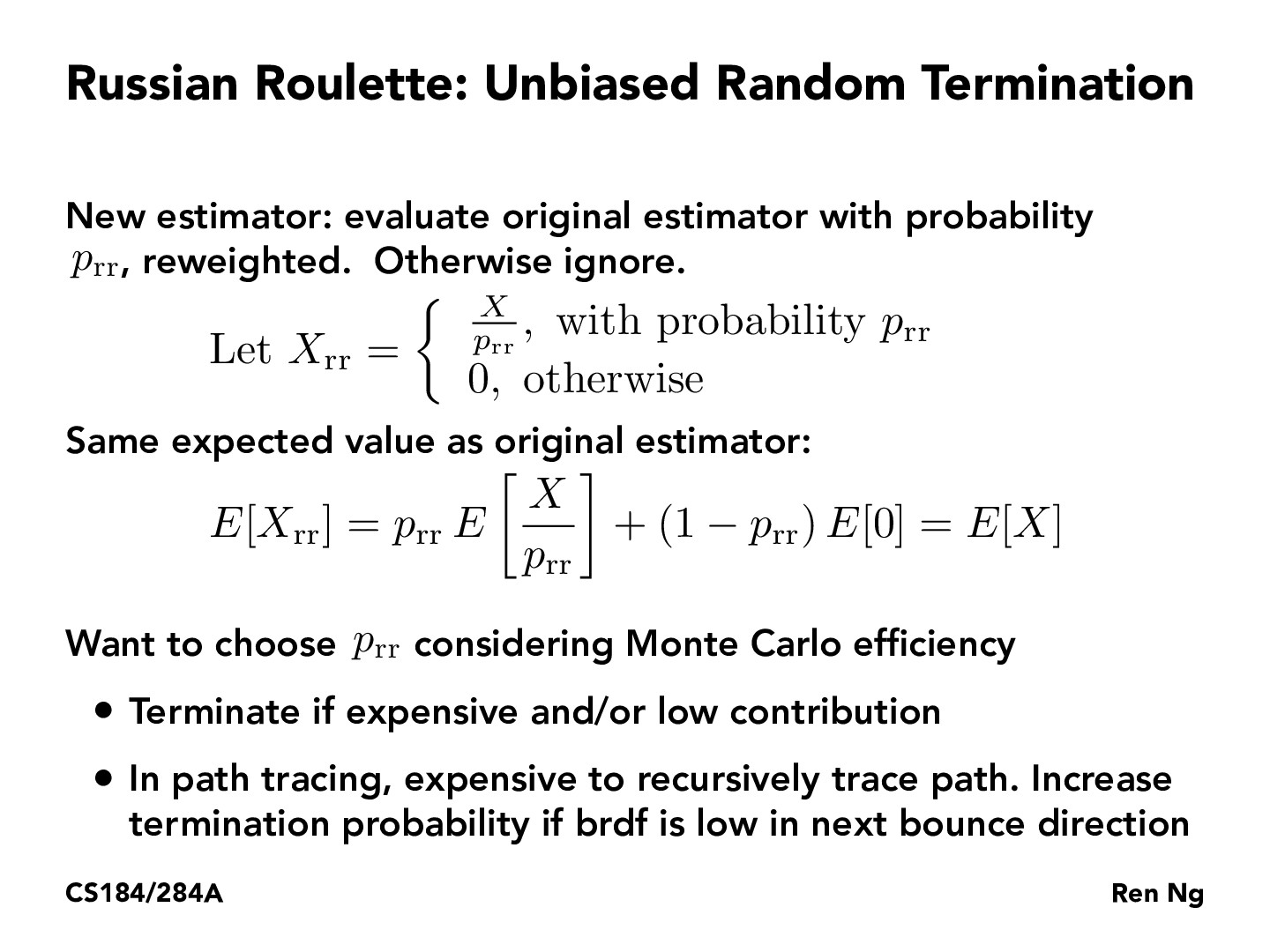You are viewing the course site for a past offering of this course. The current offering may be found here.Lecture 13: Global Illumination & Path Tracing (81)ABSchloss

Any intuition into common choices for p_rr, or is it extremely variable by scene? If there is a common choice, does it seem to model the real world well? Or is real world modeling inefficient so, if we chose solely for efficiency, we end up with a noticeably different result?bufudash

How does Russian Roulette, aka reweighing first and terminate if high expense unbiased? Isn't it still true in this case that we cannot account what happens if a light takes n+1 bounces?visatish

@bufudash I believe it is unbiased by definition because it has the same expected value as the original estimatorvisatish

@ABSchloss I looked at some example implementations online, and it seems that $p_{rr}$ is not really a constant value but dynamically computed at runtime and changes as the algorithm progressesChrisP19

Although the estimator is unbiased, I was curious about its effect on the variance:
$V[X_{rr}] = E[X_{rr}^2] - E[X_{rr}]^2$

$E[X_{rr}^2] = p_{rr} E[\frac{X^2}{p_{rr}^2}]$
$= \frac{1}{p_{rr}} E[X^2]$

$E[X_{rr}]^2 = E[X]^2$

$V[X_{rr}] = \frac{1}{p_{rr}} E[X^2] - E[X^2]$
$= V[X] + (\frac{1}{p_{rr}} - 1)E[X^2]$

Let's say $p_{rr} = \frac{1}{2^n}$, then the increase in variance scales at a rate of $2^n$. We can also note if $p_{rr}=1$, then there is no increase in variance.

You must be enrolled in the course to comment